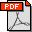Article Details

Numerical Analysis Of Non-Newtonian Rheology Effect On Hydrocyclone Flow Field

Oleh   LIN YANG [-]
 Kontributor / Dosen Pembimbing : Jia-Lin Tian ; Zhi Yang ; You Li ; Chuan-Hong Fu ; Yong-Hao Zhu ; Xiao-Lin Pang Jenis Koleksi : Jurnal elektronik Penerbit : Lain-lain Fakultas : Subjek : Engineering & allied operations Kata Kunci : Non-Newtonian rheology, Cyclone separation, Key parameters, Turbulent drag reduction, FLUENT Sumber : Petroleum , Volume 1, Issue 1, Pages 63-67 (March 2015), http://dx.doi.org/10.1016/j.petlm.2015.05.001 Staf Input/Edit : Suharsiyah   Ena Sukmana File : 1 file Tanggal Input : 2019-11-08 15:06:572015 EJRNL PP LIN YANG 1.pdf ]

Terbatas
» ITB

In view of the limitations of the existing Newton fluid effects on the vortex flow mechanism study, numerical analysis of non Newton fluid effects was presented. Using Reynolds stress turbulence model (RSM) and mixed multiphase flow model (Mixture) of FLUENT (fluid calculation software) and combined with the constitutive equation of apparent viscosity of non-Newtonian fluid, the typical non-Newtonian fluid (drilling fluid, polymer flooding sewage and crude oil as medium) and Newton flow field (water as medium) were compared by quantitative analysis. Based on the research results of water, the effects of non-Newtonian rheology on the key parameters including the combined vortex motion index n and tangential velocity were analyzed. The study shows that: non-Newtonian rheology has a great effect on tangential velocity and n value, and tangential velocity decreases with non-Newtonian increasing. The three kinds of n values (constant segment) are: 0.564(water), 0.769(polymer flooding sewage), 0.708(drilling fluid) and their variation amplitudes are larger than Newtonian fluid. The same time, non-Newtonian rheology will lead to the phenomenon of turbulent drag reduction in the vortex flow field. Compared with the existing formula calculation results shown, the calculation result of non-Newtonian rheology is most consistent with the simulation result, and the original theory has large deviations. The study provides reference for theory research of non-Newtonian cyclone separation flow field.

Pencarian Lanjut
Artikel Terkait
Info Digilib ITB
Tautan